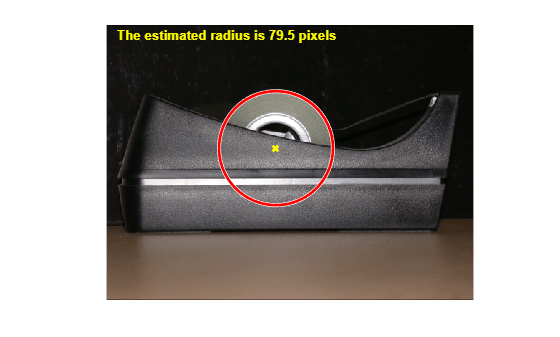## Measuring the Radius of a Roll of Tape

This example shows how to measure the radius of a roll of tape, which is partially obscured by the tape dispenser. Utilize `imfindcircles` to accomplish this task.

Read in `tape.png`.

```RGB = imread('tape.png'); imshow(RGB); hTxt = text(15,15,'Estimate radius of the roll of tape',... 'FontWeight','bold','Color','y');```### Step 2: Find the Circle

Find the center and the radius of the circle in the image using `imfindcircles`.

```Rmin = 60; Rmax = 100; [center, radius] = imfindcircles(RGB,[Rmin Rmax],'Sensitivity',0.9)```
```center = 1×2 236.9291 172.4747 ```
```radius = 79.5305 ```

### Step 3: Highlight the Circle Outline and Center

```% Display the circle viscircles(center,radius); % Display the calculated center hold on; plot(center(:,1),center(:,2),'yx','LineWidth',2); hold off; delete(hTxt); message = sprintf('The estimated radius is %2.1f pixels', radius); text(15,15,message,'Color','y','FontWeight','bold');```